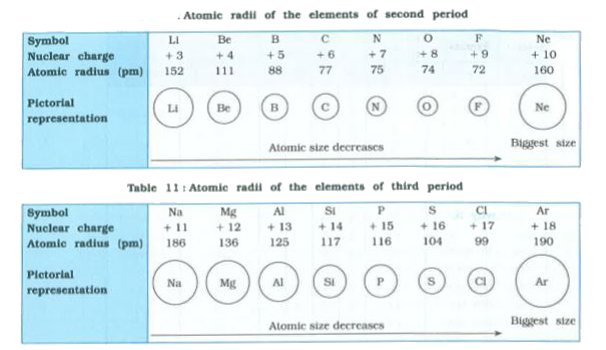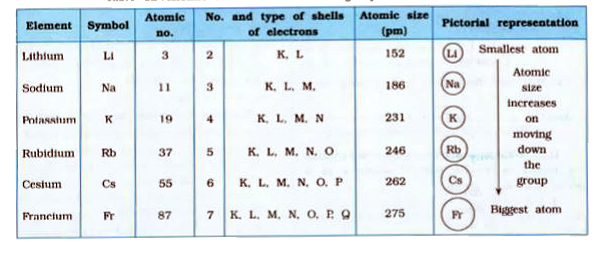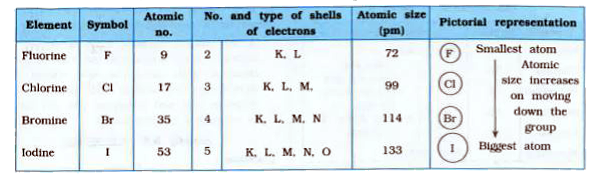# What is meant by atomic size ? Explain the trends in atomic size of elements in a period and in a group.

##### Updated On: 27-06-2022

लिखित उत्तर

Solution
If an atom is considered to be a sphere, then the term .atomic size. is described by .atomic radius..
Atomic radius : The average distance between the centre of the nucleus and the outermost shell which contains electrons in an isolated atom is called atomic radius.
Atomic radius may be expressed in angstroms (A0) or centimeter (cm) or in picometers (pm).
1A0=108cm=1010m
1pm=102m
Variation in atomic radii in a period :
On moving from left to right in a period, the atomic number of element increases successively by 1. So nuclear charge increases by 1. Due to this increased nuclear charge, the attraction between electron and nucleus increases, hence the atomic size decreases.
Thus, on moving from left to right in a period, there is a trend of decrease in atomic radii.Variation of atomic radii (atomic size) in a group:
On moving dwon a group, a new shell of electrons is added. As a result the distance between the nucleus and the valence shell increases.
In a group, the distance between the valence electron and nucleus increases with increase in nuclear charge.
Thus, on moving dwon a group, the atomic radii of elements increase gradually.